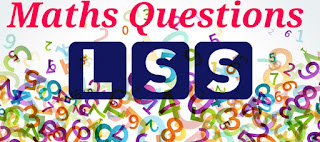# LSS Model Question - MATHS (Eng Medium) - Part 02

1Here are some maths questions for LSS Examination. It is also useful for Terminal Examinations. Please coments for errors and suggestions....
01
There was 7 two thousand rupee note, 9 five hundred rupee note, 16 hundred rupee note, 24 ten rupee note, 6 rupee note, 11 two rupee coins and 7 one rupee coins in Appu’s shop.
A] What is the total amount?
B] Biju bought things for 135 rupees from this shop. He gave a five hundred rupee note. How much will he get as balance?
C] What is the amount in the shop now?
D] How many hundred rupee notes are there in the shop now?
02
Which are the numbers that can be written usig all the digits 2, 6, 8 and 9 only once?
A] Which is the biggest number?
B] Find the difference between the largest number and the smallest number of these.

01
A] What is the total amount?
7 X 2000 = 14000
9 X 500 = 4500
16 X 100 = 1600
24 X 10 = 240
6 X 5 = 30
11 X 2 = 22
7 X 1 = 7
Total = 20399
B] Biju bought things for 135 rupees from this shop. He gave a five hundred rupee note. How much will he get as balance?
500 – 135 = 365
C] What is the amount in the shop now?
20399 + 135 = 20534
D] How many hundred rupee notes are there in the shop now?
16- 3 = 13 notes [3 notes were used for giving the balance.]
02
Which are the numbers that can be written usig all the digits 2, 6, 8 and 9 only once?
Starting with 2 :- 2689, 2698, 2869, 2896, 2968, 2986
Starting with 6 :- 6289, 6298, 6829, 6892, 6928, 6982
Starting with 8 :- 8269, 8296, 8629, 8692, 8926, 8962
Starting with 9 :- 9268, 9286, 9628, 9682, 9826, 9862
A] Which is the biggest number?
9862
B] Find the difference between the largest number and the smallest number of these.
9862 - 2689 = 7173
Tags:

1.